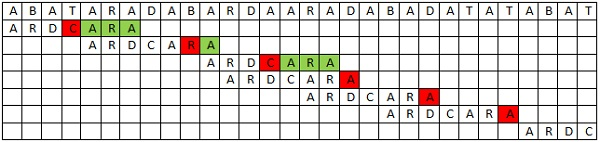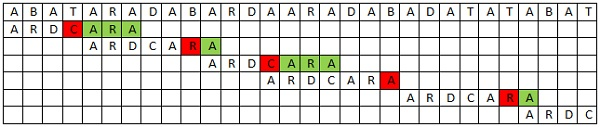## Wednesday, September 12, 2012

### Boyer Moore string Matching algorithm

Boyer Moore string matching algorithm is one of the improved version of Naive or Brute Force String Matching Algorithm. In this method we try to get more than one position movement like in Brute Force method to minimize running time of string matching process.This method use two running time heuristics to minimize running time.
1. Looking-Glass Heuristics
2. Character-Jump Heuristic
Now lets get idea of these two Heuristic

#### Looking-Glass Heuristics

There we start comparison of our pattern with the text from the end of pattern and
move backward to the front of pattern. This comparison technique may not good all the time than comparing from first letter to last letter of the pattern. Sometimes it may be better than front to the backward comparing and sometimes may not.
As an example get pattern as FOOD and comparing letters of the text as GOOD.
then front to the backward comparison get only one comparison to move to the next position. Because F and G gets mismatch. But backward to the front comparison get 4 comparisons to move to the next position. Therefore, we can't say that Looking-Glass Heuristics is not always time effective. It may be or may not be.

#### Character-Jump Heuristic

In this technique, we find more number of movement positions than moving one by one position when we get mismatches. Let text array as T[] and pattern array as P[]. If we find that T[i] letter mismatch with P[j] letter. Then we find that T[i] letter is in anywhere of P that does not take comparison in that position. If it is, then we move P as get align with the T[i] letter. If it is not,then move pattern completely past T[i] letter.

According to the above two heuristics, we can say that,
1. Looking-Glass heuristic enables to get to the destination faster by going backward if there is a mismatch during the consideration of P at a certain location in T.
2. Character-Jump heuristic enables to avoid lots of needless comparisons by significantly shifting P relative to T.
There are three types of Boyer Moore string Matching algorithms.
3. Good Suffix Rule

Mismatching character of the text aligned with the pattern is called Bad Character. In this rule we should have to find right most occurrences of each letters of the alphabet. As an example lets get
Alphabet S={A,B,C,D,R,T}
Pattern P= ARDCARA

Now lets find right most occurrence positions(R) of alphabet
R(A) = 7               R(B) = 0             R(C) = 4           R(D) = 3           R(R) = 6            R(T) = 0

ALGORITHM:
if T[k] letter mismatched with P[i] letter
shift P along by max[1,i-R(T[k])]Red Boxes - Mismatches               Green Boxes - Matches
Explanation of each raw.
1. First raw is the text and second raw is the pattern.
2. Last three letters matched and C mismatched. Bad Character is T and i is 4. Find right most occurrence of  T in Pattern. That is R(T) = 0. Then find (i- R(T) ) = 4-0 = 4. Then find max(1,4). That is 4. Then move by 4 positions.
3. Last letter matched and R mismatched. Bad Character is B and i is 6. Find right most occurrence of B in Pattern. That is R(B) = 0. Then find (i- R(B) ) = 6-0 = 6. Then find max(1,6). That is 6. Then     move by 6 positions.
4. Last three letters matched and C mismatched. Bad Character is A and i is 4. Find right most occurrence of  A in Pattern. That is R(A) = 7. Then find (i- R(A)) = 4-7 = -3. Then find max(1,-3). That is 1. Then move by 1 positions.
5. Last letter A mismatched. Bad Character is D and i is 7. Find right most occurrence of  D in Pattern. That is R(D) = 3. Then find (i- R(D) ) = 7-3 = 4. Then find max(1,4). That is 4. Then move by 4 positions.
6. Last letter A mismatched. Bad Character is D and i is 7. Find right most occurrence of  D in Pattern. That is R(D) = 3. Then find (i- R(D) ) = 7-3 = 4. Then find max(1,4). That is 4. Then move by 4 positions.
7. Last letter A mismatched. Bad Character is T and i is 7. Find right most occurrence of  T in Pattern. That is R(T) = 0. Then find (i- R(T) ) = 7-0 = 7. Then find max(1,7). That is 7. Then move by 7 positions.
Above example we can see,
We get more than one shift only when  R(T[k]) + 1 < i. Otherwise we shift the pattern by only one position. Therefore this rule is not useful when R(T[k]) >= i. Therefore we move on to extended bad character rule.

In this rule we does not consider right most occurrences of letters of alphabet and we should have to find all the occurrences of each letters of the alphabet. As an example lets get
Alphabet S={A,B,C,D,R,T}
Pattern P= ARDCARA

Now lets find  occurrence positions of alphabet
A_list = {7,5,1}            B_list = {Ø}           C_list = {4}        D_list = {3}
R_list = {2,6}             T_list = {Ø}Red Boxes - Mismatches             Green Boxes - Matches

Explanation of each raw.
1. First raw is the text and second raw is the pattern.
2. Last three letters matched and C mismatched. Bad Character is T and i is 4. View occurrence list of T. That is T_list = {Ø}.  Then move by 4 positions.
3. Last letter matched and R mismatched. Bad Character is B and i is 6. View occurrence list of B. That is B_list = {Ø}.  Then move by 6 positions.
4. Last three letters matched and C mismatched. Bad Character is A and i is 4. View occurrence list of A. That is A_list = {7,5,1}. Then find nearest less value than i of A_list. That is 1 and i is 4. Then move by (4-1)positions. That means move by 3 positions.
5. Last letter A mismatched. Bad Character is B and i is 7. View occurrence list of B. That is B_list = {Ø}.  Then move by 7 positions.
6. Last letter matched and R mismatched. Bad Character is T and i is 6. View occurrence list of T. That is T_list = {Ø}.  Then move 6 positions.
Above example we can see,
We get more than one position shift (three position) in fifth raw using extended bad character rule. But using bad character rule we get only one position shift in fifth raw. Therefore we can say that Extended Bad Character Rule is efficient that Bad Character Rule.

You can find C Source Code for Boyer Moore String Matching Algorithm using extended bad character rule  from following link.

#### Pre-processing

In above string matching algorithm using Bad character rule, there is a process done before starting string matching process. That is finding right most occurrence or finding all the occurrences of the alphabet in the pattern. This process is called as pre-processing. This process also get  running time. Pre-processing process is used to avoid (n-m+1) shifts in Naive algorithm.
Also we can see that pre-processing time is depend on the alphabet. Because we find occurrences of the alphabet in the pattern. So we can say that woks the fastest when the alphabet is moderately sized and the pattern is relatively long.

#### Running Time Analysis

Boyer Moore String matching algorithm  is not like Naive algorithm. Becouse above mentioned that this algorithm take time for pre-processing. Therefore, addition of pre-processing time and string matching time is the running time of this algorithm.
Lets get : n-length of text                  m-length of pattern                    |Σ| -length of alphabet
1. Pre-processing time                                                                                                                                     In worst case using bad character rule takes ( |Σ| + m) comparisons. Because in each letter of alphabet(|Σ|), we find right most occurrence of pattern. Pattern length is m. So that takes  ( |Σ| + m) comparisons.Therefore, running time becomes O( |Σ| + m).
2. String matching time                                                                                                                                      In worst case using bad character rule takes (mn) comparisons. Because in each letter of pattern(m) compare with text and move by one position each time, So we should have to make (n-m+1) positions. So all the comparisons becomes  ( m*(n-m+1)). Simply we say (mn) comparisons. Therefore, running time becomes O(nm).
Therefore the worst case running time of Boyer-Moore algorithm is O(nm + |∑|)

1. Boyer-Moore algorithm is extremely fast on large alphabet (relative to the length of the pattern).

1.